## High Pass Filter:

The prototype high pass filter T and π sections are as shown in the Fig. 9.9.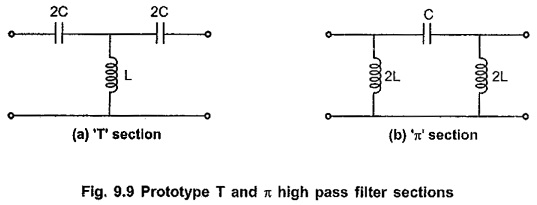### Design Impedance (R0):

Total series arm impedance Z1 = -j/ωC

Total shunt arm impedance Z2 = jωL

Hence, Z1 . Z2 =(-j/ωC) (jωL) = L/C which is real and constant. Hence above sections are constant K sections. So we can write,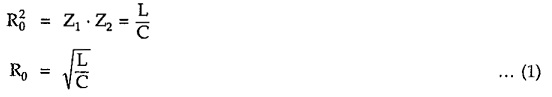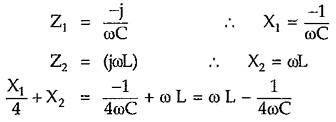#### Reactance Curves and Expression for Cut-off Frequency:

As both T and π sections have same cut-off frequency, it is sufficient to calculate the cut-off frequency for the T section only.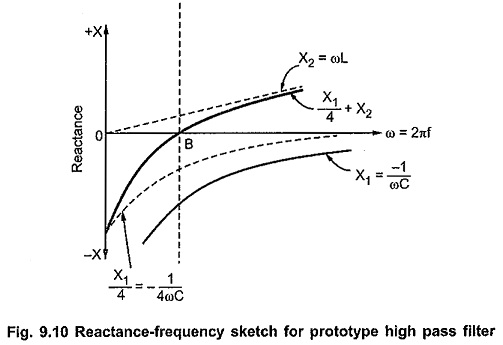The reactance curves are as shown in the Fig. 9.10.

From above characteristics it is clear that all the reactance curves have positive slope as all curves slope upward to the right side with increasing ω.

Here the curves are on the same side of the horizontal axis up to the point B, giving a stop band. For frequencies above point B, the curves are on opposite sides of the axis, giving pass band. Thus, point B gives cut-off frequency, represented as ω = ωc.

At point B, ω = ωc, the curve for (X1/4 + X2) crosses the frequency axis, hence we can write,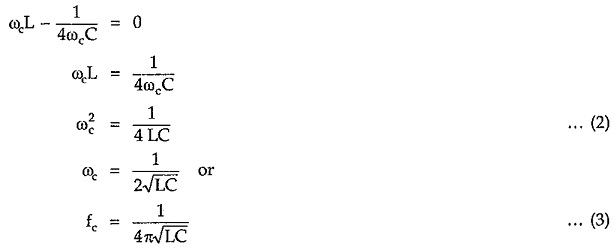The algebraic approach to calculate cut-off frequency is as follows.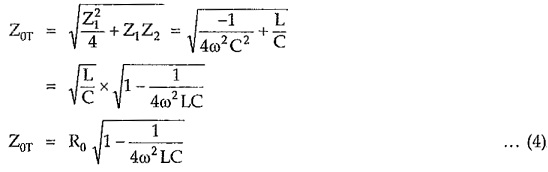From above expression it is clear that, Z0T is real if 1/4ω2LC < 1 and imaginary if 1/4ω2LC > 1. Hence condition 1 – 1/4ω2LC = 0 gives expression,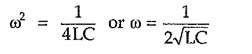Thus, above prototype section passes all frequencies above ω = 1/2√LC while attenuates all frequencies below this value. Therefore cut-off frequency of high pass filter is given by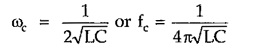Above frequency comes out to be same as frequency calculated by reactance sketch method.

#### Variation of Z0T and Z0π with Frequency:

Consider expression for Z0T as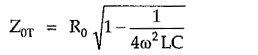From equation (2) we can write,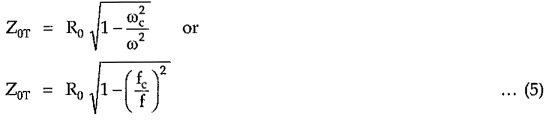Similarly we can write,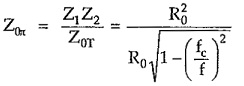Hence,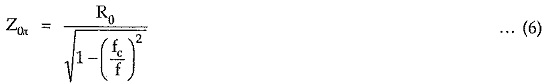From equation (5), it is clear that as frequency f increases from fc to ∞ in pass band, Z0T also increases from 0 to R0. For π section, from equation (6), it is clear that as frequency increases from fc to ∞, Z0 decreases from ∞ to R0 in pass band. The variation of Z0T and Z with frequency is as shown in Fig. 9.11.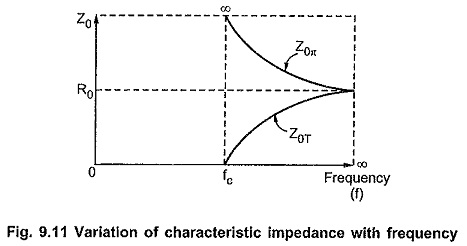#### Variation of Attenuation Constant (α) with Frequency:

In pass band, attenuation is zero (α = 0). In stop band attenuation is given by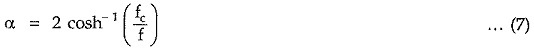In stop band, as frequency f increases from 0 to fc, attenuation decreases from ∞ to 0. The variation of attenuation constant α with frequency is as shown in the Fig. 9.12.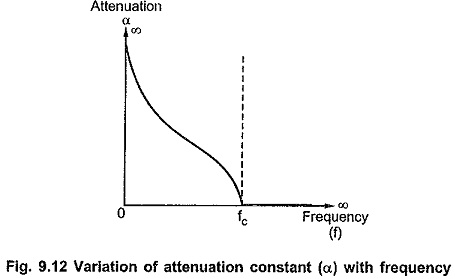#### Variation of Phase Constant β with Frequency:

In stop band, phase constant β is always π radian. In pass band where α = 0, the phase angle β is given by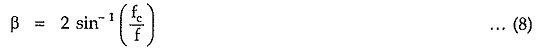From the above equation it is clear that in pass band when frequency f increases from fc to ∞, β decreases to 0. The variation of phase constant β with frequency is as shown in the Fig. 9.13.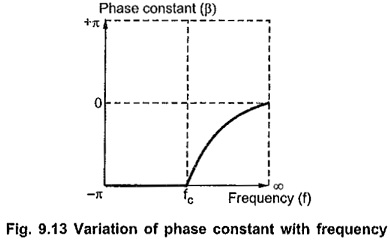#### Design Equations of Prototype High Pass Filter:

The design impedance R0 and cut-off frequency fc for high pass filter section can be given in terms of L and C as follows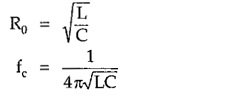Dividing equation for R0 by fc, we get,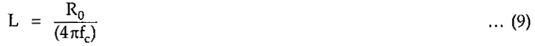Multiplying equation for R0 and fc, we get,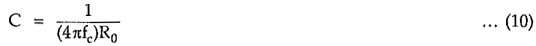Equation (9) and (10) are called design equations of prototype high pass filter sections.

Scroll to Top